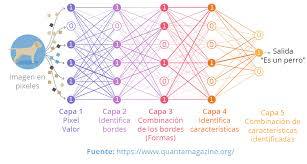DEEP LEARNING

29.04.2020

Artificial Neural Networks

The machine learning architecture was originally inspired by the biological brain (particularly the neuron) by which deep learning is carried out.

ANNs vary in their architectures quite considerably, and therefore there is no definitive neural network definition.

The 2 generally cited characteristics of all ANNs are the possession of adaptative weight sets and the capability of approximating non-linear functions of the inputs to neurons.

Softmax

Softmax activation functions are normally used in the output layer for classification problems.

It is similar to the sigmoid function, with the only difference being that the outputs are normalized, to sum up to 1.

The sigmoid function would work in case we have a binary output, however, in case we have a multi-class classification problem, softmax makes it easy to assign values to each class which can be easily interpreted as probabilities.

Perceptron

A perceptron is a simple linear binary classifier.

Perceptrons take inputs and associated weights (representing relative inputs) and combine them to produce an output, which is then used for classification.

Perceptrons have been around a long time, with early implementations dating back to the 1950s, the first of which were involved in early ANN implementations.

Multilayer Perceptron (MLP)

A multilayer perceptron (MLP) is the implementation of several fully adjacently connected layers of perceptrons, forming a simple feedforward neural network.

This multilayer perceptron has the additional benefit of nonlinear activation functions, which single perceptrons do not possess.

Activation Function

Once the linear component is applied to the input, a non-linear function is applied to it. This is done by applying the activation function to the linear combination.

The activation function translates the input signals to output signals. The output after application of the activation function would look something like f(a*W1+b) where f() is the activation function.

Dropout

Dropout is a regularization technique that prevents over-fitting of the network.

As the name suggests, during training a certain number of neurons in the hidden layer is randomly dropped. This means that the training happens on several architectures of the neural network on different combinations of the neurons.

You can think of drop out as an ensemble technique, where the output of multiple networks is then used to produce the final output.

Cost Function

When we build a network, the network tries to predict the output as close as possible to the actual value. We measure this accuracy of the network using the cost/loss function. The cost or loss function tries to penalize the network when it makes errors.

Our objective while running the network is to increase our prediction accuracy and to reduce the error, hence minimizing the cost function. The most optimized output is the one with the least value of the cost or loss function.

Learning Rate

The learning rate is defined as the amount of minimization in the cost function in each interaction.

In simple terms, the rate at which we descend towards the minima of the cost function is the learning rate.

We should choose the learning rate very carefully since it should neither be very large that the optimal solution is missed and nor should be very low that it takes forever for the network to converge.

Backpropagation

When we define a neural network, we assign random weights ad bias values to our nodes. Once we have received the output for a single iteration, we can calculate the error of the network.

This error is then fed back to the network along with the gradient of the cost function to update the weights of the network. These weights are then updated so that the errors in the subsequent iterations are reduced.

This updating of weights using the gradient of the cost function is known as back-propagation.

Source: Data Science App